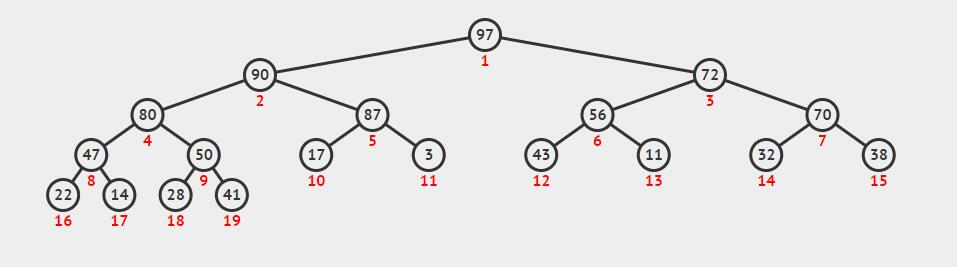# STL解析 —— heap

## Standard Template Library —— heap

ZingLix May 27, 2018

heap 又称二叉堆，是一个十分常用的数据结构，可以看成一个完全二叉树，但可以使用数组来实现。在这有过更详细的介绍。• is_heap： 判断 [first, last) 中元素是否为最大堆。
• make_heap：在 [first, last) 中构造最大堆。
• push_heap：将 last-1 中元素插入到 [first, last-1) 的最大堆中。
• pop_heap：交换在位置 first 的值和在位置 last-1 的值，并令子范围 [first, last-1) 变为最大堆。
• sort_heap：转换最大堆 [first, last) 为以升序排序的范围。

## 辅助函数

1
2
3
4
5
6
7
8
9
10
11
12
13
14
template<class Distance>
Distance _left(Distance i) {
return 2 * i + 1;
}

template<class Distance>
Distance _right(Distance i) {
return 2 * i + 2;
}

template<class Distance>
Distance _parent(Distance i) {
return (i - 1) / 2 ;
}


1
2
3
4
5
6
7
8
9
10
11
12
13
14
15
16
17
18
19
template<class RandomIt,class Distance>
void _max_heapify(RandomIt first,Distance index,Distance size) {
Distance l = _left(index),r=_right(index);
Distance largest;
if(l<size&&*(first+l)>*(first+index)) {
largest = l;
} else {
largest = index;
}
if(r<size&&*(first+r)>*(first+largest)) {
largest = r;
}
if(largest!=index) {
auto tmp = *(first + index);
*(first + index) = *(first + largest);
*(first + largest) = tmp;
_max_heapify(first, largest, size);
}
}


1
2
3
4
5
6
7
8
9
10
11
template<class RandomIt,class Distance>
void _percolate_up(RandomIt first,Distance index) {
auto value = *(first + index);
auto parent =_parent(index);
while (index!=0&& value > *(first + parent)) {
*(first + index) = *(first + parent);
index = parent;
parent = _parent(parent);
}
*(first + index) = value;
}


## push_heap

1
2
3
4
template<class RandomIt>
void push_heap(RandomIt first,RandomIt last) {
_percolate_up(first, last - first-1);
}


## pop_heap

pop_heap用于将最大的元素出堆，但实际上是将其放到最后，而原本在最后的元素需要将其调整到合适的位置。所以这一函数思路为将最前和最后的元素互换，再对新的第一个元素调用max_heapify维护堆的性质。

1
2
3
4
5
6
7
template<class RandomIt>
void pop_heap(RandomIt first, RandomIt last) {
auto tmp = *first;
*first= *(last - 1);
*(last - 1) = tmp;
_max_heapify(first, first-first, last - first-1);
}


## make_heap

max_heapify可以使某个节点满足堆性质，那么对所有节点都调用一次就可以得到最大堆。而有一半节点为叶节点，所以只用对一半节点做该操作。

1
2
3
4
5
6
7
template<class RandomIt>
void make_heap(RandomIt first, RandomIt last) {
auto size = last - first;
for(auto i=size/2;i>=0;--i) {
_max_heapify(first, i, size);
}
}


## sort_heap1
2
3
4
5
6
template<class RandomIt>
void sort_heap(RandomIt first,RandomIt last) {
while(last-first>1) {
lix::pop_heap(first, last--);
}
}


## is_heap

1
2
3
4
5
6
7
8
9
10
11
12
13
14
15
template<class RandomIt,class Distance>
bool _is_heap(RandomIt first,Distance size){
for(Distance i=0;i<size/2;++i) {
if (_left(i) < size&&*(first + i) < *(first + _left(i)))
return false;
if (_right(i) < size&& *(first + i) < *(first + _right(i)))
return  false;
}
return true;
}

template< class RandomIt >
bool is_heap(RandomIt first, RandomIt last) {
return _is_heap(first, last - first);
}